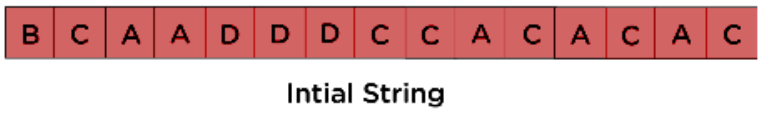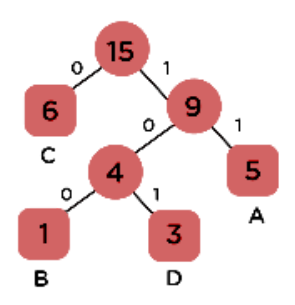×

Search anything:

#### AlgorithmsGet this book -> Problems on Array: For Interviews and Competitive Programming

1. Introduction to Huffman Coding
2. 5 Advantages of Huffman Coding
3. 5 Disadvantages of Huffman Coding
4. Comparison of Huffman Coding with other schemes
5. Conclusion

## Introduction to Huffman Coding

• Huffman coding is a form of lossless data compression that is based on the frequency of symbols in a dataset.
• It is a prefix coding scheme, which means that the encoded data does not contain any redundant bits.
• Huffman coding is widely used in various applications such as image and video compression, data transmission, and data storage.

### Example

Consider the below string:After Compression:The size table is given below:

Charactor Frequency Code Size
A 5 11 5x2=10
B 1 100 1x3=3
C 6 0 6x1=6
D 3 101 3x3=9
4x8 = 32 bits 15 bits 28 b its

Size before encoding: 120 bits
Size after encoding: 32 + 15 + 28 = 75 bits

## 5 Advantages of Huffman Coding

Following are 5 Advantages of Huffman Coding:

1. Huffman coding is an efficient method of data compression, as it assigns shorter codes to symbols that appear more frequently in the dataset. This results in a higher compression ratio.

2. Huffman coding is a prefix coding scheme, which means that it does not require any special markers to separate different codes. This makes it easy to implement and decode.

3. Huffman coding is a widely used method of data compression and is supported by many software libraries and tools, making it easy to integrate into existing systems.

4. Huffman coding is a lossless compression method, meaning the original data can be reconstructed exactly from the compressed data.

5. Huffman coding is a simple and efficient algorithm and can be easily implemented in software and hardware.

## 5 Disadvantages of Huffman Coding

Following are 5 Disadvantages of Huffman Coding:

1. Huffman coding requires the frequency of each symbol to be known in advance, making it less suitable for situations where the distribution of symbols is not known or changes dynamically.

2. Huffman trees can be complex and difficult to understand, making it harder to debug and maintain the code.

3. The coding process can be time-consuming and computationally expensive, especially for large datasets.

4. Huffman coding is not always the most efficient method of compression, and there may be other methods that provide better compression ratios for a given dataset.

5. Huffman coding can be less effective on data where there are very few unique symbols, or where the symbols are already highly compressed.

## Comparison of Huffman Coding with other schemes

Let's look at some other encoding schemes which are also used for data compression as Huffman Coding:

1. Arithmetic coding: This is a form of entropy encoding that is based on the probability of symbols in a dataset. It is more efficient than Huffman coding, but it is also more complex and difficult to implement.
2. LZW (Lempel-Ziv-Welch) encoding: This is a dictionary-based method of data compression that replaces repeating patterns of data with references to a dictionary. LZW can be faster and simpler to implement than Huffman coding.
3. Run-length encoding: This is a simple form of data compression that replaces repeating sequences of the same symbol with a single symbol and a count. It is fast and easy to implement, but its compression ratio is often low.
4. Lossy compression such as JPEG,MPEG etc. These schemes are based on the human visual perception and discard the less important information to achive high compression ratio.

In general, Huffman coding is often considered to be a good trade-off between compression efficiency and ease of implementation. Other encoding schemes may be more appropriate depending on the specific requirements of a given application.

## Conclusion

• In conclusion, Huffman coding is an efficient method of lossless data compression that is widely used in various applications.
• However, it has its own set of disadvantages that need to be taken into consideration before using it.
• It's important to consider the specific requirements of a given application and choose the most appropriate compression method accordingly.